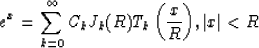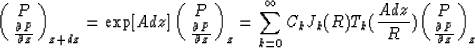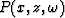Next: GENERATION OF SURFACE VALUES Up: THE GENERALIZED PHASE-SHIFT METHOD Previous: Arbitrary velocity structures

## Tal-Ezer Method

When the velocity structure varies arbitrarily in the lateral direction, the eigenvalues and eigenvectors of matrix A can no longer be obtained by inspection. It would therefore seem that a matrix diagonalization would have to be performed before each propagation to equation (11). However, recent work by Tal-Ezer(1986) indicates how the calculation of matrix exponential can be done without having to resort to expensive matrix diagonalizations. The solution is based on a Chebychev expansion for the function ex given by(18)
where x is imaginary and C0=1 and Ck=2 for k>0. The polynomials Tk(x) satisfy the recurrence relations

T0(x) = 1,

T1(x) = x,

and

Tk+1(x)=Tk-1(x)+2xTk(x)

(Tal-Ezer, 1986). By analogy with equation (18), the exponent in equation (4) is expanded according to(19)
This expansion is valid when the eigenvalues of [Adz] are purely imaginary and R must be chosen large enough to span the range of the eigenvalues of [Adz]. It was shown in Tal-Ezer(1986) that for k > R, the series expansion converges exponentially. The number of terms required in the sum in equation (19) will therefore always be finite. Equation (19) serves as the basis for implementing the generalized phase-shift migration. First the range R of the eigenvalues of [Adz] need to be estimated. Based on the case of laterally uniform velocity, Kosloff showed the estimate r=wdz/cmin with cmin denoting the lowest velocity in the strip (z,z+dz), is sufficient for stable results. The Bessel functions Jk(R) are computed next. The solutionis then calculated recursively according to following: The first two values of T0, T1 needed to initialize the recursion are given byandThe next polynomial is generated by the recursion formulaThese steps are repeated until a sufficient number of terms have been calculated in the sum(19). Then the solution is carried out in the next lower level. Whenhas been calculated completely, the final migrated section is cumulated by(Kosloff and Baysal, 1983).Next: GENERATION OF SURFACE VALUES Up: THE GENERALIZED PHASE-SHIFT METHOD Previous: Arbitrary velocity structures
Stanford Exploration Project
1/13/1998